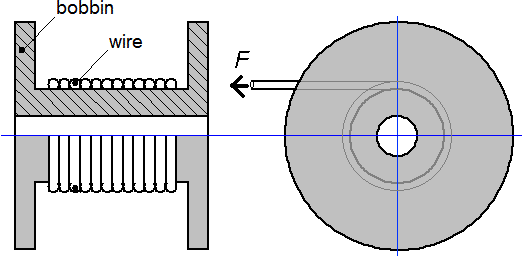QuickField

A new approach to field modelling
 Language: Global English Deutch Espanol Francais Italiano Danmark Ceske Chinese Pycckuü

Main >> Applications >> Sample problems >> Bobbin deformation caused by the winding of wiring

# Bobbin deformation caused by winding force of wiring

Problem Type:
Axisymmetric problem of stress analysis.

Geometry:Given:
Winding force, F = 50 N.

Calculate the bobbin deformation caused by the winding force.

Solution:

Let's consider the winding process. Winding force leads to a wire elongation. After the force is removed the wire shrinks back and squeezes the bobbin.
The similar effect may be achieved by cooling down the loose wiring. To simulate wire shrink it is convenient to use temperature-based elongation effect.

Relative elongation of the wire according to the Hooke's law is

dL/L0 = F / (E·Sw), where
E - Young modulus, Pa;
F - Force, N;
Sw - cross section of the wire, m2;
L0 - initial length of the wire, m.

Relative elongation of the wire according to the thermal expansion law is

dL/L0 = α·dT, where
α - coefficient of the thermal expansion, 1/K;
dT - temperature difference, K.

Hence the temperature difference (that would cause the same wire elongation as by mechanical winding force):

dT = 1/α · F/(E·Sw)

We apply this temperature difference to the loose wire to simulate the wire shrink pressure.Result:

Calculation of the bobbin deformation in QuickField• Video: